# Graph Analytics using Virtuoso’s SPARQL-BI extensions to SPARQL

## Graph Analytics using Virtuoso’s SPARQL-BI extensions to SPARQL

This post demonstrates how a combination of SQL (one open standard) with SPARQL (another open standard), here comprising a Business Intelligence-focused extension of SPARQL we call SPARQL-BI, can address a range of data manipulation operations commonly referred to as Graph Analytics.

Virtuoso’s SQL engine includes a Transitivity extension that’s surfaced in its SPARQL engine as part of the SPARQL-BI extensions to SPARQL. This functionality lets SPARQL be used for Graph Analytics operations.

### What is a Graph?

A Graph is a pictorial — a graphic aid — for representing Entity Relationship Types, i.e., an Entity and its Characteristics.

This kind of pictorial comprises a collection of nodes and connectors that represent —

• an Entity
• an Entity Relationship Type Name (a/k/a Attribute)
• an Entity Relationship Type Value (a/k/a Value)

In the RDF Entity Relationships model, propositions are represented using sentences/statements structured as subject→predicate→object. Thus, an RDF graph comprises —

• a Subject (corresponding to the Entity, above)
• a Predicate (corresponding to the Attribute, above)
• an Object (corresponding to the Value, above)

### What is a Network?

A sub-category of a Graph that depicts Entity Relationship Types that are transitive in nature i.e., all the nodes in the graph represent relatives albeit by varying degrees. Examples of popular networks include Facebook, and Twitter.

#### Social Network

This form of network is constructed from “knows” (as exemplified by FOAF), “friend” (as exemplified by Facebook) and “follows” (as exemplified by Twitter) connections that denote transitive entity relationship types.

Here’s an illustration of a Social Network constructed using terms from the FOAF Vocabulary, where each `foaf:knows` connection denotes an instance of an `owl:TranstiveProperty` and `owl:ObjectProperty` (i.e., a Property type that has a Reference [e.g., a Hyperlink] as its value).

#### Friend Network

In this kind of network each `:friend` connection denotes a transitive relationship type that’s also symmetric in nature i.e., instance of an `owl:ObjectProperty`, `owl:TransitiveProperty`, and `owl:SymmetricProperty` — signified by the bidirectional arrows indicating the mutual nature of friendship.

Naturally, you can use Reasoning and Inference (which underlie the notion of “Artificial Intelligence”) to generate a Friend Network from a Social Network. That will be the subject of another post in this series.

### What is the difference between a Graph and a Network?

Graphs are generic Entity Relationship Type (Relations) depictions while Networks are Graphs that depict Relations that are at the very least transitive in nature i.e., nodes depict relatives by varying degrees of relatedness.

### Graph Analytics

Graph Analytics are used to extract information from Entity Relationship Types as part of a query processing pipeline aimed at providing a variety of insights into the power and value of nodes within a network.

#### Why are Graph Analytics important?

Entity Relationships model the real world and provide a powerful basis for knowledge acquisition and dissemination, leveraging the productivity offered by computer software.

#### How do I apply Graph Analytics to a Knowledge Graph deployed as a Semantic Web comprising Linked Data?

This kind of Knowledge Graph is deployed as a collection of RDF sentences that adheres to Linked Data principles, meaning —

• Each Entity and Entity Relationship Type is identified unambiguously using a hyperlink (specifically, an HTTP URI)
• Entities and Entity Relationship Types are described using RDF sentence collections

Here are the steps for creating a Sociality-oriented Knowledge Graph deployed as a Semantic Web:

1. Describe the Social Network — i.e., create an RDF document comprising sentences that describe the social network’s membership
2. Load the RDF document to a Virtuoso instance — e.g., sponge (import) the document using our URIBurner service
3. Write your queries using SPARQL-BI
5. Share your Query Results pages with others via your internal network or the World Wide Web

### Social Network Graph Analytics Examples

For the live examples that follow, I will be using a FOAF-based Social Network, described using RDF-Turtle Notation.

The Dataset below is loaded to a Named Graph using a SPARQL 1.1 `INSERT` command.

#### Linear Representation of Data

``````# FOAF Social Network for Graph Analytics
# using SPARQL-BI

PREFIX foaf: <http://xmlns.com/foaf/0.1/>
WITH <urn:analytics>
INSERT

{
## Turtle Start ##

<urn:a> foaf:knows <urn:b> .
<urn:a> foaf:knows <urn:c> .
<urn:a> foaf:knows <urn:d> .
<urn:a> foaf:knows <urn:e> .
<urn:a> foaf:knows <urn:f> .
<urn:a> foaf:knows <urn:m> .
<urn:a> foaf:knows <urn:g> .
<urn:a> rdfs:label "a" .
<urn:b> foaf:knows <urn:c> .
<urn:b> foaf:knows <urn:a> .
<urn:b> rdfs:label "b" .
<urn:c> foaf:knows <urn:a> .
<urn:c> foaf:knows <urn:b> .
<urn:c> foaf:knows <urn:i> .
<urn:c> foaf:knows <urn:l> .
<urn:c> rdfs:label "c" .
<urn:d> foaf:knows <urn:k> .
<urn:d> foaf:knows <urn:a> .
<urn:d> rdfs:label "d" .
<urn:e> foaf:knows <urn:m> .
<urn:e> foaf:knows <urn:a> .
<urn:e> rdfs:label "e" .
<urn:f> foaf:knows <urn:a> .
<urn:f> foaf:knows <urn:i> .
<urn:f> rdfs:label "f" .
<urn:g> foaf:knows <urn:h> .
<urn:g> foaf:knows <urn:j> .
<urn:g> foaf:knows <urn:k> .
<urn:g> foaf:knows <urn:m> .
<urn:g> foaf:knows <urn:a> .
<urn:g> rdfs:label "g" .
<urn:h> foaf:knows <urn:g> .
<urn:h> rdfs:label "h" .
<urn:i> foaf:knows <urn:c> .
<urn:i> foaf:knows <urn:f> .
<urn:i> rdfs:label "i" .
<urn:j> foaf:knows <urn:g> .
<urn:j> rdfs:label "j" .
<urn:k> foaf:knows <urn:d> .
<urn:k> foaf:knows <urn:g> .
<urn:k> rdfs:label "k" .
<urn:l> foaf:knows <urn:c> .
<urn:l> rdfs:label "l" .
<urn:m> foaf:knows <urn:g> .
<urn:m> foaf:knows <urn:e> .
<urn:m> foaf:knows <urn:a> .
<urn:m> rdfs:label "m" .

## Turtle End ##
}
``````

#### Graphic Representation of FOAF-based Social Network Data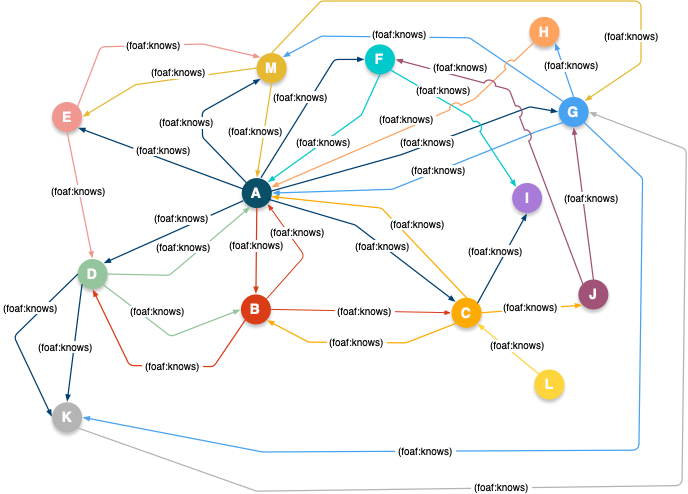#### Centrality Query

Centrality measures the importance of a node in a graph simply by how “central” the node is, relative to others in the same graph.

``````PREFIX foaf: <http://xmlns.com/foaf/0.1/>
SELECT                  ?s
( COUNT ( * ) AS ?cnt )
FROM <urn:analytics>
WHERE
{ ?s foaf:knows ?o }
GROUP BY ?s
ORDER BY ( ?cnt )
``````
##### Query Results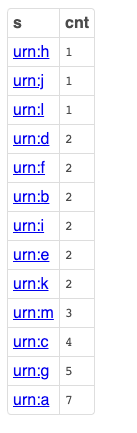#### Degree Centrality Query

Measures network importance based on in-bound connections from other nodes in the graph, or out-bound connections to other nodes in the graph, given a starting node.

``````PREFIX foaf: <http://xmlns.com/foaf/0.1/>

SELECT            ?s
( ?dist AS ?dist_to_o )
?dist_to_via
?via
?dist_from_via
?o
FROM <urn:analytics>
WHERE
{
?s foaf:knows ?o
OPTION ( TRANSITIVE ,
T_DISTINCT ,
T_IN ( ?s ) ,
T_OUT ( ?o ) ,
T_MIN ( 1 ) ,
T_MAX ( 100 ) ,
T_STEP ( 'step_no' ) AS ?dist
) .
?o foaf:knows ?via
OPTION ( TRANSITIVE ,
T_DISTINCT ,
T_IN ( ?o ) ,
T_OUT ( ?via ) ,
T_MIN ( 1 ) ,
T_MAX ( 100 ) ,
T_STEP ( 'step_no' ) AS ?dist_to_via
) .
?via foaf:knows ?s
OPTION ( TRANSITIVE ,
T_DISTINCT ,
T_IN ( ?via ) ,
T_OUT ( ?s ) ,
T_MIN ( 1 ) ,
T_MAX ( 100 ) ,
T_STEP ( 'step_no' ) AS ?dist_from_via
) .
FILTER ( ?s = <urn:a> )
FILTER ( ?dist = ( ?dist_to_via + ?dist_from_via ) )
}
``````
##### Query Results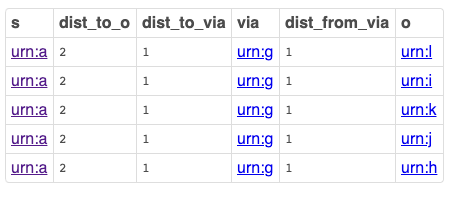#### Closeness (Reach or Distance) Centrality Query

Closeness Centrality is based on the number of “hops” (sometimes counted as the number of intervening nodes plus one) between two nodes, based on the shortest path between each pair of nodes.

``````PREFIX foaf: <http://xmlns.com/foaf/0.1/>

SELECT                    ?s
?o
( SUM ( ?dist ) AS ?sum )
FROM <urn:analytics>
WHERE
{
?s foaf:knows ?o
OPTION ( TRANSITIVE ,
T_DISTINCT ,
T_SHORTEST_ONLY ,
T_IN ( ?s ) ,
T_OUT ( ?o ) ,
T_MIN ( 1 ) ,
T_STEP ( 'step_no' ) AS ?dist
) .
FILTER ( ?s = <urn:a> )
}
GROUP BY ?s ?o
``````
##### Query Results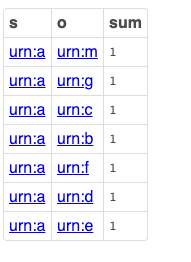#### Betweenness Centrality Query

Betweenness Centrality assigns importance to a node in a network based upon the number of times that node occurs within the paths between all pairs of nodes in a graph. That is, each node in the graph is paired with every other node; the shortest path between each pair of nodes is computed; and then the number of occurrences of each intermediate node in those shortest-paths is counted, to discover their relative importance.

This is a node intermediacy metric, in a nutshell.

``````PREFIX foaf: <http://xmlns.com/foaf/0.1/>

SELECT ?s
?o
?via
?dist
?dist_to_via
?dist_from_via
FROM <urn:analytics>
WHERE
{
?s foaf:knows ?o
OPTION ( TRANSITIVE ,
T_DISTINCT ,
T_IN ( ?s ) ,
T_OUT ( ?o ) ,
T_MIN ( 1 ) ,
T_MAX ( 100 ) ,
T_STEP ( 'step_no' ) AS ?dist
) .
?o foaf:knows ?via
OPTION ( TRANSITIVE ,
T_DISTINCT ,
T_IN ( ?o ) ,
T_OUT ( ?via ) ,
T_MIN ( 1 ) ,
T_MAX ( 100 ) ,
T_STEP ( 'step_no' ) AS ?dist_to_via
) .
?via foaf:knows ?s
OPTION ( TRANSITIVE ,
T_DISTINCT ,
T_IN ( ?via ) ,
T_OUT ( ?s ) ,
T_MIN ( 1 ) ,
T_MAX ( 100 ) ,
T_STEP ( 'step_no' ) AS ?dist_from_via
) .
FILTER ( ?s = <urn:a> )
FILTER ( ?dist = ( ?dist_to_via + ?dist_from_via ) )
}
GROUP BY ?s ?o ?via ?dist ?dist_to_via ?dist_from_via
ORDER BY ?dist
``````
##### Query Results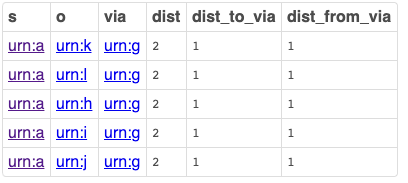#### Betweenness Centrality using Shortest Path

In this metric the shortest path is explicitly sought when determining the intermediacy metric.

``````PREFIX foaf: <http://xmlns.com/foaf/0.1/>

SELECT ?s
?via
?o
?dist_to_via
?dist_from_via
FROM <urn:analytics>
WHERE
{
?s foaf:knows ?via
OPTION ( TRANSITIVE ,
T_DISTINCT ,
T_IN ( ?s ) ,
T_OUT ( ?via ) ,
T_MIN ( 1 ) ,
T_STEP ( 'step_no' ) AS ?dist_to_via
) .
?via foaf:knows ?o
OPTION ( TRANSITIVE ,
T_SHORTEST_ONLY , ## Shortest Path Option
T_DISTINCT ,
T_IN ( ?via ) ,
T_OUT ( ?o ) ,
T_MIN ( 1 ) ,
T_STEP ( 'step_no' ) AS ?dist_from_via
) .
FILTER ( ?s = <urn:a> )
}
ORDER BY ?s ?dist_to_via
``````

#### Betweenness Centrality using out-link threshold via `T_MAX()`

In this intermediacy metric calculation, we continue to focus on the shortest path, and move the focus to out-bound rather than in-bound links.

``````PREFIX foaf: <http://xmlns.com/foaf/0.1/>

SELECT                  ?via
( COUNT ( * ) AS ?cnt )
FROM <urn:analytics>
WHERE
{
?s foaf:knows ?via
OPTION ( TRANSITIVE ,
T_DISTINCT ,
T_IN (?s) ,
T_OUT (?via) ,
T_MIN (1) ,
T_MAX(10) ,
T_STEP ('step_no') AS ?dist_to_via
) .
?via foaf:knows ?o
OPTION ( TRANSITIVE ,
T_SHORTEST_ONLY ,
T_DISTINCT ,
T_IN (?via) ,
T_OUT (?o) ,
T_MIN (1) ,
T_STEP ('step_no') AS ?dist_from_via
) .
FILTER ( ?s = <urn:a> )
}
GROUP BY ?via
ORDER BY DESC (?cnt)
``````

#### Eigen Vector Centrality (EVC)

The Eigen Vector Centrality (EVC) metric measures the importance of a node in a graph based on the the importance of its neighbors. For instance, in a Social Network, the nature of an Influencer depends on the number of people they influence — where influence depends on the total size of their audience rather than individual connection count, i.e., rather than catering to a Star-Network with many unconnected low-audience followers, you end up with an Influence-Network comprising a few nodes that each connect to a large-audience follower.

Here, we leverage Virtuoso’s built-in `evc_score` function (built using a Stored Procedure).

``````PREFIX foaf: <http://xmlns.com/foaf/0.1/>

SELECT                          ?s
( sql:EVCS ( ?M, ?s ) AS ?evc )
WHERE
{
GRAPH ?g { ?s ?p ?o }
FILTER (  ?g = <urn:analytics>
&& ?p = foaf:knows
)
BIND ( sql:EVC_MATRIX ( ?g , ?p ) AS ?M )
}
GROUP BY ?s
ORDER BY DESC ( ?evc )
``````
##### Query Results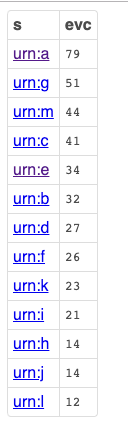### Conclusion

Contrary to general misconceptions about the capabilities of the SQL and SPARQL open standards, you can apply the power of both of these query languages to the challenge of Graph Analytics. Even better, you can do so in a manner that preserves your ability to mix and match “best of class” products, so long as they adhere to such open standards.

### Related

Using Twitter as my source of data to construct a Social Network, here are SPARQL examples using common Network (Graph) Analytics algorithms.

## Centrality

``````SELECT               ?o
( COUNT(*) AS ?followersCount )
FROM <urn:tweets:kg>
WHERE { ?s opltw:follows ?o }
GROUP BY ?o
ORDER BY DESC ( ?followersCount )
LIMIT 100
``````

## Degree Centrality

``````## follower (@asemeota) is 2-degrees of separation from the
## followees, i.e., he doesn't follow any of them directly

PREFIX foaf: <http://xmlns.com/foaf/0.1/>
SELECT ?s as ?follower
?o as ?followee
( SUM ( ?dist ) AS ?degrees )
WHERE
{
?s  opltw:follows  ?o
OPTION (
TRANSITIVE ,
T_DISTINCT ,
T_SHORTEST_ONLY ,
T_IN ( ?s ) ,
T_OUT ( ?o ) ,
T_MIN ( 2 ) ,
T_STEP ( 'step_no' ) AS ?dist
)
FILTER ( ?s = <https://twitter.com/asemota#this> )
}
GROUP BY ?s ?o
``````

## Precomputed S-Rank (a variant of Eigen Vector Centrality)

``````PREFIX custom-rank: <LONG::>

SELECT                                                    ?s AS ?startNode
sql:rnk_scale ( SUM ( custom-rank:IRI_RANK ( ?s ) ) ) AS ?startNodeRank
?o AS ?endNode
sql:rnk_scale ( SUM ( custom-rank:IRI_RANK ( ?o ) ) ) AS ?endNodeRank